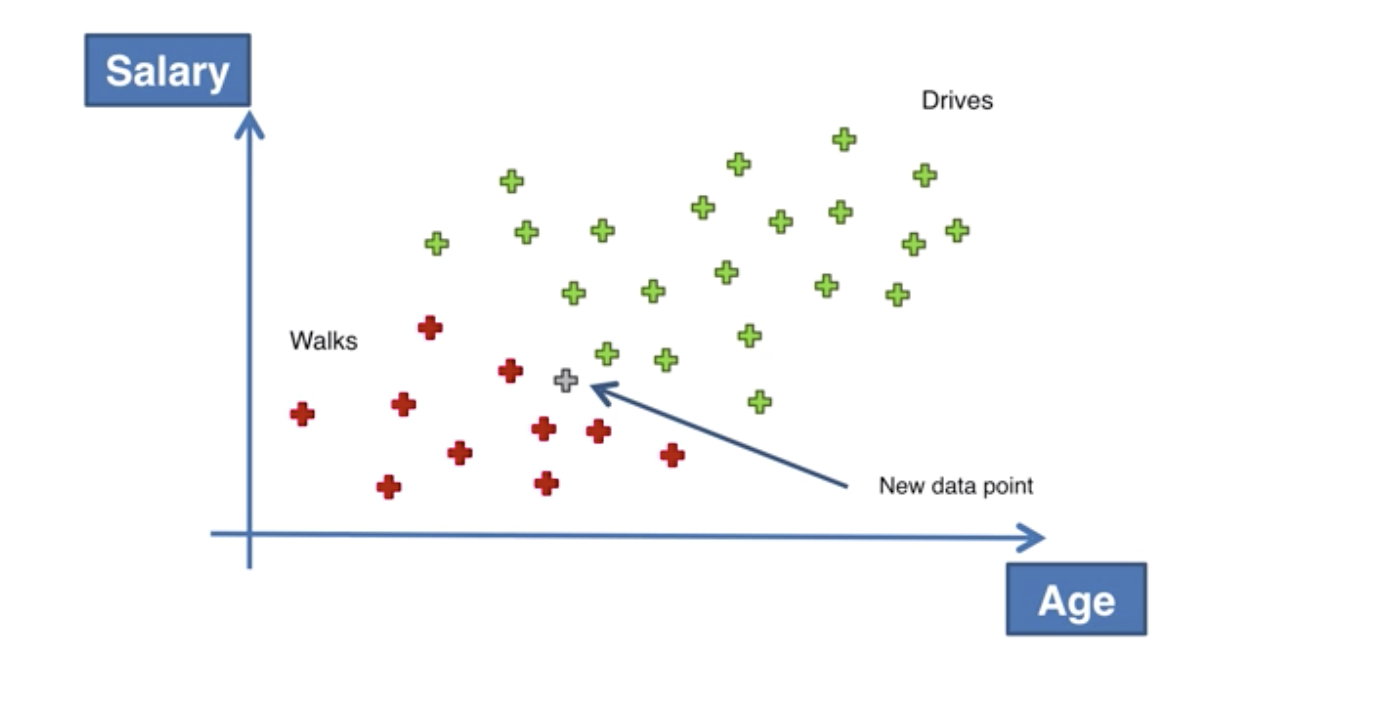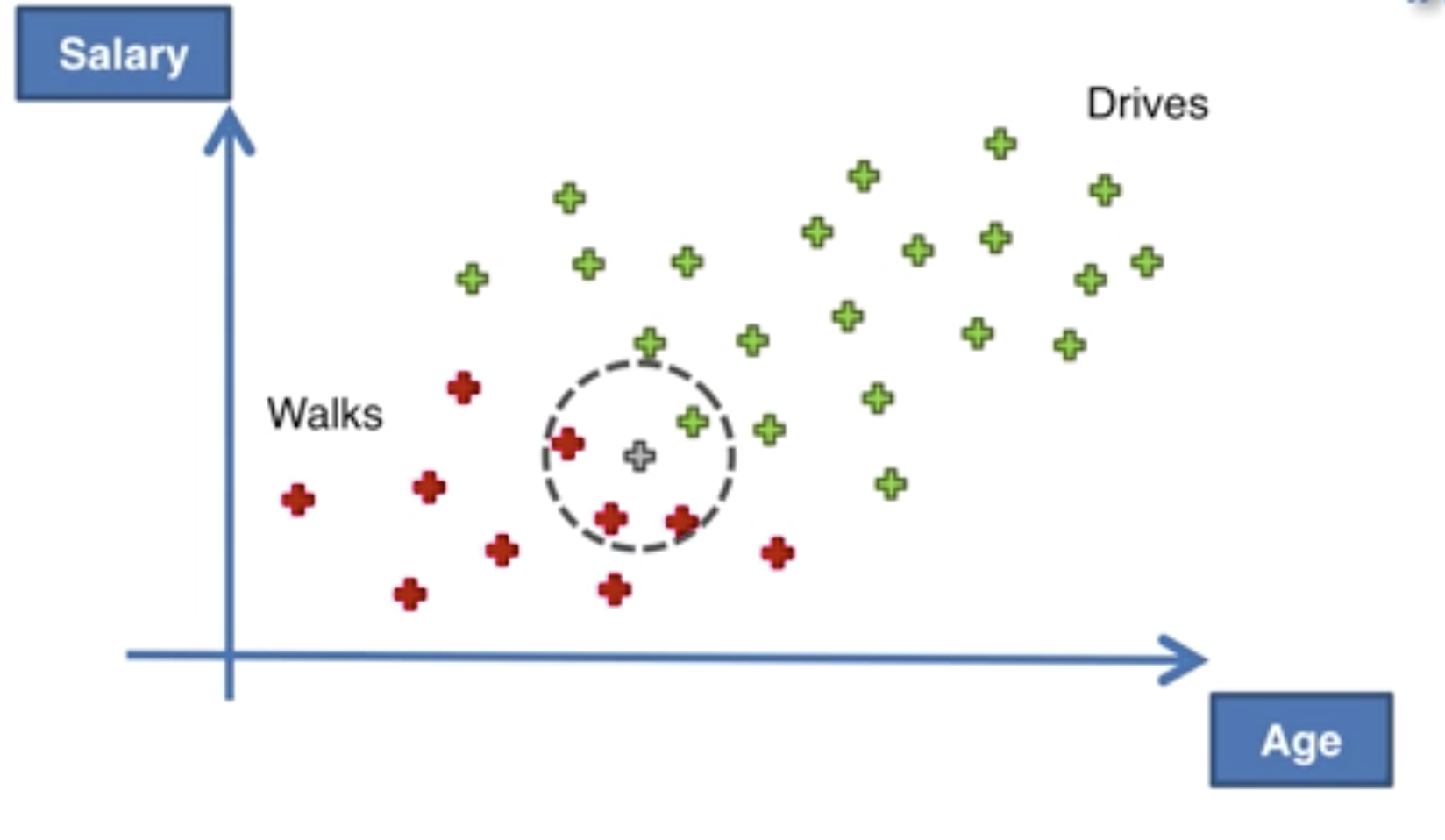## 贝叶斯定理

P(A|B) = \frac{P(B|A)*P(A)}{P(B)}

• 1号机器每小时生产30个扳手
• 2号机器每小时生产30个扳手
• 所有的产品中有1%的次品
• 所有次品中有50%来自机器1，50%来自机器2

P(Defect|Mach2) = \frac{P(Mach2|Defect)_P(Defect)}{P(Mach2)}

## 朴素贝叶斯P(Walks|X) = \frac{P(X|Walks)*P(Walks)}{P(X)}

P(Drives|X) = \frac{P(X|Drives)*P(Drives)}{P(X)}## 代码实现

from sklearn.naive_bayes import GaussianNB
classifier = GaussianNB()
classifier.fit(X_train, y_train)